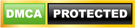Blog

• Home
• PHP
• Conditions and Loops in PHP

# Conditions and Loops in PHP

• ###### | 1836 Ratings

CONSTANT

DEFINE:-

Define is the pre-defined key-word in php. This will take two arguments. The first argument being the constant identifier which identifier its second argument value. We can pass third argument as Boolean to make case in-sensitiveCheckout PHP Interview Questions

CONDITIONS

Conditional statements are used to perform different actions based on different conditions.

IF CONDITION:-WHILE CONDITION:-

The while loop executes a block of code while a condition is trueOUTPUT:-

The number is 1
The number is 2
The number is 3
The number is 4
The number is 5

Related Page: Cookies, Sessions And Buffer In PHP

DO-WHILE CONDITION:-

The do…while statement will always execute the block of code once, it will then check the condition, and repeat the loop while the condition is true.Example

The example below defines a loop that starts with i=1. It will then increment i with 1, and write some output. Then the condition is checked, and the loop will continue to run as long as i is less than, or equal to 5:FOR LOOP:-

Loops execute a block of code a specified number of times, or while a specified condition is true. The for loop is used when you know in advance how many times the script should run.

FOR EACH:-

for each is usefull for all non-sequence data of an array. Associative array ,numerical array object data of an array
The general syntax is
foreach (arrayName as arr[value]))
{
echo arr [value];
}
Another syntax is
foreach (arrayname as key=>value)
{
echo key’-‘value         (or)        echo “key-value”;   }

Ex: -foreach.php